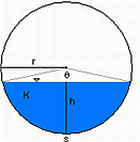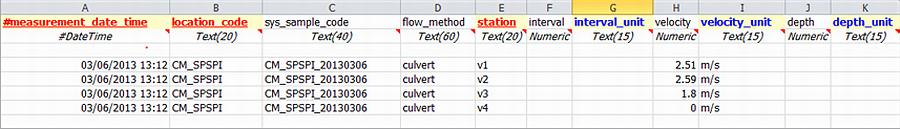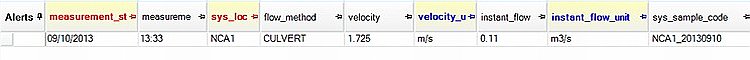﻿ Culvert Flow Method

# Culvert Flow Method

Navigation:  EDGE > EDGE Features > Flow Calculations >

# Culvert Flow Method

This method calculates the instant flow (m3/sec) within a culvert at a given location from field measurements based on the Culvert Flow Method.Schematic Cross-Section of a Culvert

where:            h = height of water in the culvert (m)

r = radius of culvert (or width/2) (m)

Ø = 2 * arcos ((r-h)/r)

The method uses the following equation:

Equation C. i)           X = Area (m2) x Velocity (m/sec)

where:           X = instant flow (m3/sec)

Area = (r2 (Ø - sin Ø)) / 2  (m2)

1.Open EDGE or the EDD. In the Flow Measurement tab, the MEASUREMENT_DATE_TIME and LOCATION_CODE (SYS_LOC_CODE) and STATION are required fields. In order to perform the instant flow calculation, the SYS_SAMPLE_CODE, FLOW_METHOD and VELOCITY (with units) columns need to be populated. The SYS_SAMPLE_CODE should be identical for all measurements relating to a specific culvert flow location/time. The FLOW_METHOD should be entered as/set to CULVERT.

2.In the Flow tab, the MEASUREMENT_START_DATE and LOCATION_CODE are required fields. To perform the calculation, the SYS_SAMPLE_CODE, FLOW_METHOD, culvert DEPTH (depth of water in the culvert) and WIDTH are also required, together with their respective units.

3.Open EDGE or the EDD.In the When opening the EDD in EDP, EDP will find corresponding Flow and Flow Measurement rows with matching SYS_SAMPLE_CODES and FLOW_METHOD, which includes the text "CULVERT".

4.If data has only been entered in Flow Measurement (not "Flow'), then EDP will create a new row in Flow Measurement, adding an average velocity to FLOW.VELOCITY.

5.If a row exists in Flow, with the culvert depth and width populated, then EDP will perform the instant flow calculation and the FLOW.INSTANT_FLOW column will be populated.

6.During the Create step in EDP, the format will create samples, populating DT_SAMPLE and DT_FIELD_SAMPLE.Example of Flow Measurement Tab in Flow EDD for the Culvert Method

7.In EDGE, the data should be entered in the Flow and Flow Measurement tabs as described above. EDGE will perform the FLOW.INSTANT_FLOW calculation on saving the EDD (the EDD may need to be re-opened to see the calculation).Example of Flow Tab in EDGE with Calculated INSTANT_FLOW value